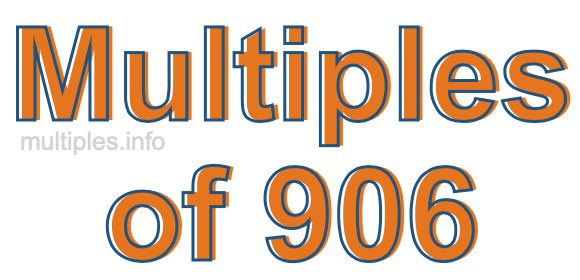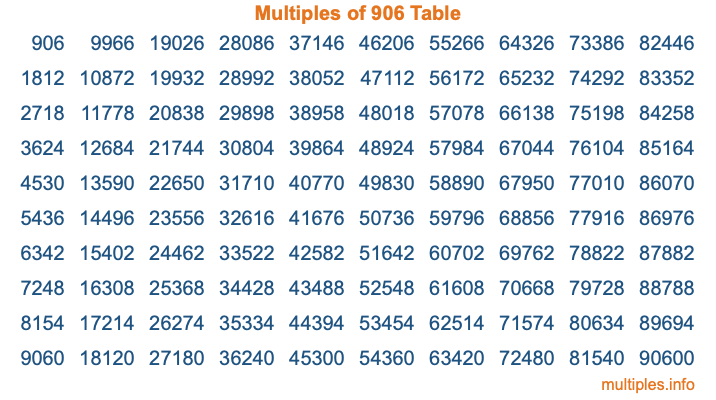Multiples of 906Welcome to the Multiples of 906 page. Here we will first teach you everything you will ever need to know about the multiples of 906, and then give you a study guide summary of everything we taught you to make sure you remember it all. Use this page to look up facts and learn information about the multiples of 906. This page will make you a multiples of nine hundred six expert!

Definition of Multiples of 906
Multiples of 906 are all the numbers that when divided by 906 equal an integer. Each of the multiples of 906 are called a multiple. A multiple of 906 is created by multiplying 906 by an integer.

Therefore, to create a list of multiples of 906, you start with 1 multiplied by 906, then 2 multiplied by 906, then 3 multiplied by 906, and so on for as long as you want. Thus, the list of the first five multiples of 906 is 906, 1812, 2718, 3624, and 4530. To see a larger list of multiples of 906, see the printable image of Multiples of 906 further down on this page. We also have a category where you can choose any nth multiple of 906.

Multiples of 906 Checker
The Multiples of 906 Checker below checks to see if any number of your choice is a multiple of 906. In other words, it checks to see if there is any number (integer) that when multiplied by 906 will equal your number. To do that, we divide your number by 906. If the the quotient is an integer, then your number is a multiple of 906.

Is  a multiple of 906?

Least Common Multiple of 906 and ...
A Least Common Multiple (LCM) is the lowest multiple that two or more numbers have in common. This is also called the smallest common multiple or lowest common multiple and is useful to know when you are adding our subtracting fractions. Enter one or more numbers below (906 is already entered) to find the LCM.

Check out our LCM Calculator if you need more details about the Least Common Multiple or if you need the LCM for different numbers for adding and subtraction fractions.

nth Multiple of 906
As we stated above, 906 is the first multiple of 906, 1812 is the second multiple of 906, 2718 is the third multiple of 906, and so on. Enter a number below to find the nth multiple of 906.

th multiple of 906

Multiples of 906 vs Factors of 906
906 is a multiple of 906 and a factor of 906, but that is where the similarities end. All postive multiples of 906 are 906 or greater than 906. All positive factors of 906 are 906 or less than 906.

Below is the beginning list of multiples of 906 and the factors of 906 so you can compare:

Multiples of 906: 906, 1812, 2718, 3624, 4530, etc.

Factors of 906: 1, 2, 3, 6, 151, 302, 453, 906

As you can see, the multiples of 906 are all the numbers that you can divide by 906 to get a whole number. The factors of 906, on the other hand, are all the whole numbers that you can multiply by another whole number to get 906.

It's also interesting to note that if a number (x) is a factor of 906, then 906 will also be a multiple of that number (x).

Multiples of 906 vs Divisors of 906
The divisors of 906 are all the integers that 906 can be divided by evenly. Below is a list of the divisors of 906.

Divisors of 906: 1, 2, 3, 6, 151, 302, 453, 906

The interesting thing to note here is that if you take any multiple of 906 and divide it by a divisor of 906, you will see that the quotient is an integer.

Multiples of 906 Table
Below is an image of the first 100 multiples of 906 in a table. The table is in chronological order, column by column. The first column has the first ten multiples of 906, the second column has the next ten multiples of 906, and so on.The Multiples of 906 Table is also referred to as the 906 Times Table or Times Table of 906. You are welcome to print out our table for your studies.

Negative Multiples of 906
Although not often discussed or needed in math, it is worth mentioning that you can make a list of negative multiples of 906 by multiplying 906 by -1, then by -2, then by -3, and so on, to get the following list of negative multiples of 906:

-906, -1812, -2718, -3624, -4530, etc.

Multiples of 906 Summary
Below is a summary of important Multiples of 906 facts that we have discussed on this page. To retain the knowledge on this page, we recommend that you read through the summary and explain to yourself or a study partner why they hold true.

There are an infinite number of multiples of 906.

A multiple of 906 divided by 906 will equal a whole number.

906 divided by a factor of 906 equals a divisor of 906.

The nth multiple of 906 is n times 906.

The largest factor of 906 is equal to the first positive multiple of 906.

906 is a multiple of every factor of 906.

906 is a multiple of 906.

A multiple of 906 divided by a divisor of 906 equals an integer.

906 divided by a divisor of 906 equals a factor of 906.

Any integer times 906 will equal a multiple of 906.

Multiples of a Number
Here you can get the multiples of another number, all with the same attention to detail as we did for multiples of 906 on this page.

Multiples of
Multiples of 907
Did you find our page about multiples of nine hundred six educational? Do you want more knowledge? Check out the multiples of the next number on our list!